﻿ Methodology of the Choice of Optimum Parameters of Technological Process on Experimental to DataPublications are Open
Access in this journal
Article Versions
Export Article
• Normal Style
• MLA Style
• APA Style
• Chicago Style
Research Article
Open Access Peer-reviewed

### Methodology of the Choice of Optimum Parameters of Technological Process on Experimental to Data

Yu. K. Mashunin
American Journal of Modeling and Optimization. 2019, 7(1), 20-28. DOI: 10.12691/ajmo-7-1-4
Received June 17, 2019; Revised July 21, 2019; Accepted July 26, 2019

### Abstract

The article presents information modeling of the technological process based on vector optimization methods, which were developed in the author’s early works. As a test example, the technology “to predict and select the best welding parameters for hybrid laser arc welding (HLAW)” was used, “to predict and select the best welding parameters during the hybrid arc welding (HLAW)”, which was published in DOI:10.12691/ajmo-6-1-2. The mathematical model of the technological process is constructed as a vector problem of mathematical programming. In the model, criteria (characteristics of the technological process) are formed under certainty conditions (the functional dependence of each characteristic and restrictions on parameters is known) and in conditions of uncertainty (there is not enough information about the functional dependence of each characteristic on parameters). We formed a test case in which the initial parameters correspond to the parameters from DOI:10.12691/ajmo-6-1-2. The modeling methodology is shown in a numerical example of a technological process with two parameters and three characteristics. The constructed vector nonlinear programming problem is implemented in the MATLAB system.

### 1. Introduction

Currently, the problem of designing technical systems, technological processes, materials using information technologies and optimization has received much attention, both in Russia and abroad 1, 2, 3, 4, 5, 6, 7, 8, 9, 10, 11, 12, 13, 14, 15. In works 2, 3, 4, 5 at the design stage, the construction of mathematical models of technical systems and their modeling based on the theory and vector optimization methods is shown. Information statement of technological process, the bound to welding of metals is presented in article 1. Using the experimental data 1 the research of technological process is conducted. The purpose of this work is to present the methodology for the selection of the optimal parameters of the technological process according to experimental data. The model is represented by a vector problem mathematical programming. The solution of the vector problem is carried out under certainty and uncertainty. To achieve this goal in the work presented: The analysis of the initial data of the technological process presented in 1 was carried out. For such a technological process, we have formed a test case and built a mathematical model, similar to the technical system in 2, 3, 4, 5; The model of the technological process is presented as a vector (multicriteria) problem of mathematical programming. Outputs characteristics of the process were presented as a polynomial of the second degree; The methodology is illustrated on the numerical example of model of the technological process with two parameters and three characteristics in the conditions of a certainty and uncertainty. The problem of nonlinear programming realized in the MATLAB system 14.

### 2. Analysis and Mathematical Formulation of the Problem of Choosing the Best Parameters of the Technological Process

2.1. Analysis of Input Data of the Technological Process

Your in work 1 the research of a number of technologies is conducted and the methodology of the choice of the best parameters of technological process according to the experimental data is presented. Consider one of the technologies “Hybrid Laser Arc Welding (HLAW)”, 1. Alloy ZE41-T5 was chosen as the material to be welded with AZ61 alloy as a filler material. The nominal composition of the alloy ZE41-T5 and AZ61Alloys (wt. the %) is presented in Table 1, 1. The experiments were planned using a three-factor central composite structure and were carried out in various welding conditions — the “parameters”: 1) laser power; 2) travel speed; 3) wire feed speed; 4) current; 5) frequency. To predict performance characteristics (functions), the following (output) characteristics were investigated: 1) weld depth; 2) under fill; 3) percentage defect; 4) total accumulated pore length. The experimental parameters of the input and output of the weld for HLAW ZE41-T5 with AZ61 filler are presented in Table 1. (Table 3A in 1).

According to its structure, Table 1 can be considered as a technical system, the study of which was carried out in 1, 2. The model of the technological process is presented as a vector (multi-criteria) problem of mathematical programming, similarly to 4, 5, 8, 9.

Output characteristics of the process were presented as a polynomial of the second degree. Similarly, we tried to present the output characteristics of Table 1 as polynomials in MATLAB. But the resulting regression matrix is not correct. We sorted the source data of Table 1 by parameters and presented them as Table 2.

From Table 2 it can be seen that in the experimental data presented for the same input parameters, the values of the output characteristics (functions) are different.

2.2. Vector Problem of Mathematical Programming, as a Mathematical Model for the Selection of Optimal Parameters of the Technological Process

The mathematical model of the technological process by analogy with the technical system 2, 3, 4, 5, 7, 8, 9, 10, 11 is investigated with conditions of certainty and uncertainty. Conditions of a certainty are characterized by the fact that the functional dependence of each characteristic and restrictions on the parameters of the technological process is known, 2, 7, 10. The conditions of uncertainty are characterized by the fact that the initial data characterizing the technological process are presented: a) random, b) fuzzy, or, c) incomplete data. Therefore, we lack sufficient information about the functional dependence of each characteristic and restrictions on the parameters 3, 8, 11. For options a) and b) basic data have to be transformed to option c) and are presented in the table form. In work the option c) - with not full data which are, as a rule, obtained from experimental data is investigated. In real life, the conditions of certainty and uncertainty are combined. The process model should also reflect these conditions. We will present a model of the technological process under certainty and uncertainty in the aggregate: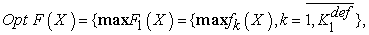(1)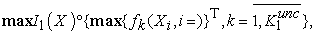(2)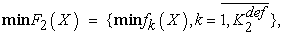(3)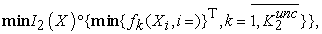(4)

at restriction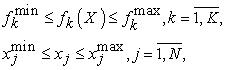(5)

where Х={xj, j =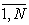} is the vector of controlled variables (input parameters of the technological process); F(X)={F1(X) F2(X) I1(X), I2(X)} is a vector criterion, each component of which represents a vector of criteria (output characteristics of the technological process). The magnitude of the characteristic (function) depends on the discrete values of the vector of variables X. F1(X), F2(X) is the set of functions max and min, respectively; I1(X) and I2(X) are the set of discrete values of the characteristics max and min, respectively; K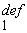, K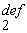(definiteness), K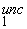, K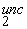(uncertainty) a set of criteria max and min formed under certainty and uncertainty; in (5), f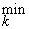fk(X)f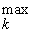, k=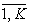is a vector-function of restrictions imposed on the operation of the technological process, x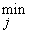xjx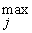, j =are parametric restrictions imposed on the technological process 12.

### 3. Methodology of the Choice of Optimum Parameters of Technological Process According to the Experimental Data

The methodology includes a number of stages. 1. Formation of the technical specifications (source data) for numerical modeling and the choice of optimum parameters of the technological process. Input data are formed by the designer, who investigates and projects the technological process. 2. Construction of a mathematical (numerical) model of the technological process in the conditions of certainty and uncertainty. 3. The solution of the vector problem of mathematical programming (VPMP) - a model of the technological process with equivalent criteria, i.e. the choice of the optimal parameters of the technological process according to experimental data with equivalent criteria. 4. Building a geometric interpretation of the results of the solution in a three-dimensional coordinate system in the relative units. 5. The solution of the vector problem of mathematical programming - a model of the technological process for a given priority of the criterion, i.e. the choice of optimal parameters of the technological process according to experimental data for a given priority of the criterion. 6. Geometric interpretation of the results of the solution in a three-dimensional coordinate system in physical units. We study a technological process for which data are known about a certain set of functional characteristics (certainty conditions), discrete values of characteristics (uncertainty condition) and restrictions imposed on the functioning of the technological process 3, 4, 5, 6. The numerical problem of modeling a technological process is solved with equivalent criteria and with a given priority of the criterion.

3.1. Stage 1. The Technical Assignment: "The choice of the optimal parameters of the technological process"

It is given. The technological process, the operation of which is determined by two parameters X={x1, x2} - the vector of variables (controlled). The operation of the process is determined by three characteristics (criteria) F(X)={f1(X), f2(X), f3(X)}, the value of which depends on the vector of parameters Х. Conditions of certainty. In this test example, there is no certainty condition. The solution to this problem can be found in 2, 4, 8, 10. Conditions of uncertainty. For the first, second and third characteristics of the technological process, the results of experimental data are known: the values of the parameters and the corresponding characteristics. The numerical values of the parameters X and the characteristics y1(X), y2(X), y3(X) are presented in Table 3. (Compare with the data in Table 2).

In the decision taken, the evaluation value for the first, second and third characteristics (criteria) are desirable to get as high as possible: f1(X)max, y2(X)max, y3(X)max. The parameters X={x1, x2} vary within the following limits: 2.0 ≤ x1 ≤ 3.5, 12.0 ≤ x2 ≤ 30.0. It is required. Build a model of the technological process in the form of a vector problem. Solve the vector problem with equivalent criteria. Choose a priority criterion. Set the numerical value of the priority criterion. Make the best (optimal) solution. Note. The author has developed software for four parameters: X={x1, x2, x3, x4} and six characteristics F(X)={f1(X), … , f6(X)}. For each task, the program is configured individually. If desired, the author can increase the number of parameters to five: X={x1, … , x5}. In the model, criteria with uncertainty conditions can vary from zero to six.

3.2. Stage 2. Construction of a Mathematical Model of the Technological Process
3.2.1. Construction in the Conditions of a Certainty

The functional dependence of each characteristic and restrictions on the technological process parameters is defined. In our example, such characteristics are absent.

3.2.2. Construction in the Conditions of an Uncertainty

Construction in the conditions of an uncertainty consists in using qualitative and quantitative descriptions of a technological process obtained on the basis of the “input-output” principle in Table 3. Converting information (initial data y1(X), y2(X), y3(X)) into a functional form f1(X), f2(X), f3(X) is carried out by using mathematical methods (the regression analysis). The initial data of Table 3 are formed as a matrix I in the MATLAB system: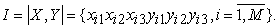(6)

For each experimental data set yk, k=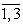the regression function is constructed using the least squares method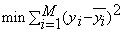in the MATLAB system. For this, the polynomial Ak is formed, which determines the interrelation of the parameters Xi ={x1i, x2i} (6) and the function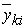= f(Xik), k=.The result is a system of coefficients Ak={A0k, A1k, …, A9k}, which determine the coefficients of the polynomial (function):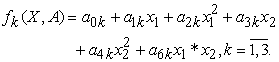(7)

The experimental data I1={xi1 xi2 yi1} of the matrix (6) are converted into the function f1(X), which, taking into account the obtained coefficients, takes the form: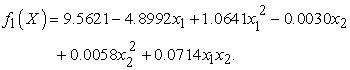(8)

The experimental data I2={xi1 xi2 yi2} of the matrix (6) are converted into the function f2(X), which, taking into account the obtained coefficients, takes the form: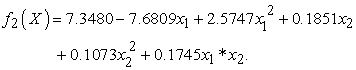(9)

The experimental data I3 = {xi1 xi2 yi3} of the matrix (6) are converted to the function f3(X), which, taking into account the obtained coefficients, takes the form: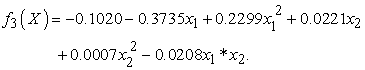(10)

3.2.3. Construction of a Mathematical Model of the Technological Process

(General part for the conditions of certainty and uncertainty). To build a mathematical model of a technological process, we use: the functions obtained by the conditions of certainty and uncertainty (8), (9), (10); parametrical restrictions.

Functions (8), (9), (10) are considered as criteria determining the purposefulness of the operation of the technological process. All criteria K = 3 are aimed at maximization: f1(X) , f2(X), f3(X) →max. As a result, the model of the functioning of the technological process will be represented by the vector problem of mathematical programming: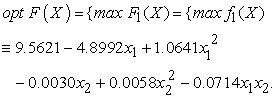(11)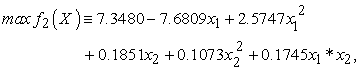(12)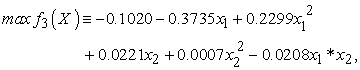(13)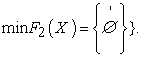(14)

at restriction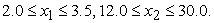(15)

The vector problem of mathematical programming (11) - (15) represents the model of optimal decision making under certainty and uncertainty in the aggregate.

3.3. Stage 3. The Solution of the Vector Problem of Mathematical Programming - the Model of the Technological Process

(Algorithm 1 for solving a vector optimization problem with equivalent criteria, 11). The solution of the vector problem (11) - (15) with equivalent criteria is represented as a sequence of steps.

3.3.1. Step 1. Solving Problem (11) - (15) For Each Criterion Separately

Solving problem (11) - (15) for each criterion separately, using the fmincon (...) function of the MATLAB system, the call to the fmincon (...) function is considered in 10. As a result of the calculation for each criterion, we obtain optimum points: X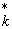and f= fk(X), k=K - the values of the criteria at this point, that is, the best solution for each criterion: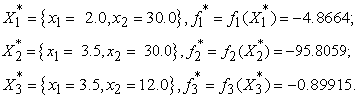(16)

The constraints (15) and optimum points (16) in the coordinates {x1, x2} are shown in Figure 1.

3.3.2. Step 2. The Worst Unchanging Part of Each Criterion is Determined

The entire document should be in times New Roman font size 10. Paper title must be centered, bold, regular font size 20 and all with upper case. at restriction.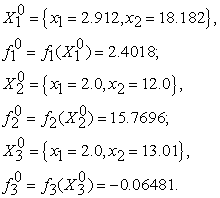(17)
• Figure 1. Pareto set, SoS in two-dimensional coordinate system

3.3.3. Step 3. A Systematic Analysis of the Set of Pareto Optimal Points

A systematic analysis of the set of Pareto optimal points (i.e. analysis by each criterion) is performed. At the optimum points X *={X1*, X2*, X3 *}, the values of the objective functions F(X*)=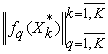are determined, the vector D=(d1 d2 d3)T deviations for each criterion on the admissible set S: dk =fk*-fk0, k=, and the relative estimation matrix: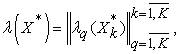where λk(X)=(fk*-fk0)/dk.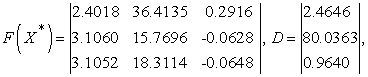(18)

where at restriction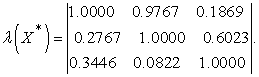(19)

The analysis of the values of criteria (18) in relative estimates (19) shows that at the points of optimum X *={X1*, X2*, X3*} (diagonally) the relative estimate is equal to one. The remaining criteria are significantly less than one. It is required to find a point (parameters) at which the relative estimates are closest to unity. The solution of this problem is aimed at solving the λ-problem - step 4.

3.3.4. Step 4. The Construction of the λ-problem

The construction of the λ-problem is carried out in two stages: a maximin optimization problem with normalized criteria is initially constructed: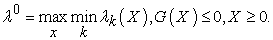(20)

At the second stage, the maximin problem (20) is transformed into a standard mathematical programming problem (λ -problem):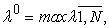(21)

at restriction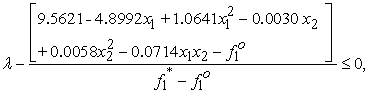(22)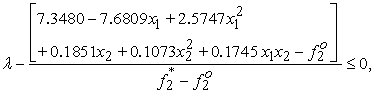(23)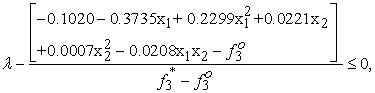(24)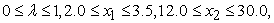(25)

where the vector of unknowns has dimension of N+1: X={x1, … , xN, λ}, N=2.

3.3.5. Step 5. Solution of the λ-problem

For this purpose we use the function fmincon(…), 13: [Xo,Lo]=fmincon('Z_TS_2Krit_L',X0,Ao,bo,Aeq,beq,lbo,ubo,'Z_TS_LConst',options). As a result of the solution of VZMP (11)-(15) at equivalent criteria and λ-problem corresponding to it (21)-(25) received: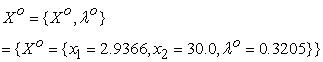(26)

optimum point, which determines the design parameters of the technological process. Point Xo is shown in Figure 1;fk(Xo), k=- values of criteria (characteristics of the technological process):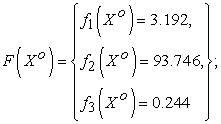(27)

λk(Xo), k=- values of relative estimates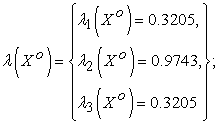(28)

λo =0.3205 is the maximum lower level among all relative estimates, measured in the relative units: λo=min1(Xo), λ2(Xo), λ3(Xo)) = 0.3205, λo - also called a guaranteed result in the relative units, i.e., λk(Xo) and, accordingly, the characteristics of the technological process fk(Xo it is impossible to improve, without worsening at the same time other characteristics.

Note that, in accordance with Theorem 2 11, at point Xo, criteria 1 and 3 are contradictory. This contradiction is determined by the equality λ1(Xo)= λ3(Xo) = λo = 0.3205, and the remaining criteria by the inequality {2(Xo)= 0.9743}>λo.

Thus, Theorem 2 serves as the basis for determining the correctness of the solution of the vector problem. In the vector problem of mathematical programming, as a rule, for two criteria, equality is satisfied: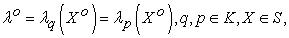(29)

and for other criteria it is defined as inequality: {λ2(Xo)}>λo.

3.4. Stage 4. Creation of Geometrical Interpretation of Results of the Decision in a Three-dimensional Coordinate

In the allowable set of points S formed by constraints (25), the optimum points X1*, X2*, X3*, which are shown in Figure 1, combined into a contour, represent the set of Pareto optimal points, So S. The coordinates of these points, as well as the characteristics of the technological process in relative units λ1(X), λ2(X), λ3(X) are shown in Figure 2 in the three-dimensional space x1, x2 and λ, where the third axis is the relative estimate λ.

• Figure 2. Geometric interpretation of the solution of the λ-problem coordinate x1, x3 and λ
3.5. Stage 5. Decision-making on the Basis of Technological Process Model at the Set Priority of Criterion

(Algorithm 2 of the decision in problems of vector optimization with a criterion priority, 11).

3.5.1. Step 1. We Solve a Vector Problem with Equivalent Criteria

The algorithm of the decision is presented in the stage 3. Numerical results of the solution of the vector problem are given above. Pareto's great number of So ⊂ S lies between optimum points X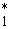X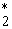X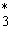X, Figure 1. This information is the basis for further research on the structure of the Pareto set. The decision maker is usually the technological process designer. If results of the solution of a vector problem with equivalent criteria do not satisfy the person making the decision, then the choice of an optimal solution is carried out from any subset of points of S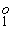, S, S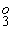.

3.5.2. Step 2. Choice of Priority Criterion of qK

From the theory (the Theorem 2 11) it is known that in an optimum point of Xo there are always two most contradictory criteria: qK and vK for which in the relative unit’s precise equality is carried out: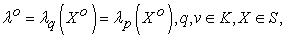and for the others it is carried out inequalities: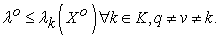For the choice of the priority criterion on the display the message about results of the solution of λ-problem in physical and relative units is given: Criteria (27) in Xo optimum point:

F(Xo)={f1(Xo)=3.192, f2(Xo)=93.75, f3(Xo)=0.2441}; The relative estimates (28) in Xo:

λ(Xo)={λ1(Xo)=0.3205, λ2(Xo)=0.971, λ3(Xo)= 0.3205}. From the function λ(Xo) it is clear that the first and the third are the most contradictory criteria: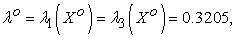(29)

Select from Figure 2 the first and third criteria and present in the relative units in Figure 3.

• Figure 3. Result the solution of the problem (21)-(25) in the three-dimensional coordinate system {x1, x3, λ}: λ1(X) and λ3(X)

As a rule, from a pair of the contradictory criteria, a criterion chosen by the decision maker would be improved. Such criterion is called "priority criterion", we will designate it q=3K. This criterion is investigated in interaction with the first criterion of k=1∈K.

On the display the message is given:

q=input ('Enter priority criterion (number) of q =') - Entered: q=3.

3.5.3. Step 3. Numerical Limits of the Change of the Size of a Priority of the Criterion

For priority criterion of q=3K changes of the numerical limits in the physical units upon transition from Xo optimum point to the point of X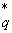received on the first step at equivalent criteria are defined. q=3 given about criterion are given for the screen: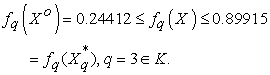(30)

In the relative units the criterion of q=3 changes in the following limits: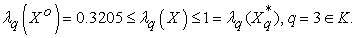This data is analyzed.

3.5.4. Step 4. Choice of Size of the Priority Criterion (Decision - making)

On the message: "Enter the size of priority criterion fq=" - we enter, the size of the characteristic: fq=0.7. f q(Xo) = 0.24412fq=0.70.89915 = fq(X), q=3K.

3.5.5. Step 4. The Relative Assessment Is Calculated

For the chosen size of priority criterion fq=0.7 the relative assessment is calculated: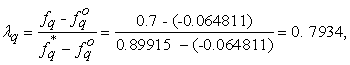(31)

which upon transition from Xo point to Xlies in the limits: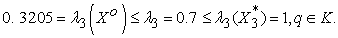3.5.6. Step 6. Let's Calculate Coefficient of the Linear Approximation

Assuming the linear nature of the change of the criterion of fq(X) in (30) and according to the relative assessment of λq, using standard linear approximation techniques, we will calculate the proportionality coefficient between λq(Xo), λq which we will call ρ: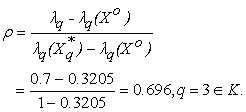(32)

3.5.7. Step 7. Let's Calculate Coordinates of a Priority of the Criteria

Assuming the linear nature of change of a vector of Xq={x1 x3}, q=3 we will determine point coordinates with dimension of fq =0.7, the relative assessment (31):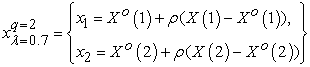where X o={x1= 2.9366, x2= 30.0}, X={x1=3.5, x2=12.0}.

As result of the decision (33) we will receive Xq point with coordinates: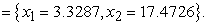(33)

3.5.8. Step 7. Calculation of the Main Indexes of a Point of Xq

For the received Xq point, we will calculate:

Ÿ all criteria in the physical units fk(xq)={fk(xq), k=}, f(Xq)={f1(xq)= 2.6107, f2(xq)= 36.1524, f3(xq)= 0.5919};

Ÿ all relative estimates with the criterion priority λq ={λ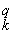, k=}, λk(xq)=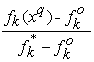, k=, k(xq)={1(xq) = 0.0847, 2(xq) = 0.2547, 3(xq) = 0.6813};

Ÿ minimum relative assessment: min(λk(Xq)) = 0.0847;

Ÿ vector of priorities of the third criterion over other criteria Pq={p=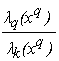, k=}: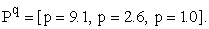Any point from Pareto's set X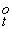={, X} So can be similarly calculated.

3.5.9. Analysis of Results

The calculated size of criterion fq(X), qK is usually not equal to the set fq. The error of the choice of Δfq=|fq(X) - fq| =|0.5919 - 0.7|=0.1081 is defined by an error of linear approximation, Δfq%= 13.5%. In the course of the modeling parametrical restrictions (20) can be changed, i.e. some set of optimum decisions is received. Choose a final version which in our example included from this set of optimum decisions:

Ÿ parameters of technological process Xo={x1= 2.9366, x2= 30.0};

Ÿ the parameters of the technical system at a given priority criterion q=3: Xq={x1= 3.3287, x2=17.4726}.

3.6. Stage 6. Geometrical Interpretation of Results of the Decision in Three to a Measured Frame in Physical Units

We represent these parameters in a two-dimensional x1, x2 and three dimensional coordinate system x1, x2 and λ in Figure 1, Figure 2, Figure 3, and also in physical units for each function f1(X), f2(X), f3(X) on Figure 4, Figure 5, Figure 6, respectively. The first characteristic f1(X) in physical units show in Figure 4.

• Figure 4. Result the solution of the problem (11)-(15) in the three-dimensional coordinate system {x1, x3 and f1(X)}

The characteristic f2(X) in physical units show in Figure 5.

• Figure 5. Result the solution of the problem (11)-(15) in the three-dimensional coordinate system {x1, x3 and f2(X)}

The third characteristic f3(X) in physical units show in Figure 6.

• Figure 6. Result the solution of the problem (11)-(15) in the three-dimensional coordinate system {x1, x3 and f3(X)}

### 4. Conclusion

The problem of making the optimal decision in a complex technological process for a certain set of functional characteristics is one of the most important problems of system analysis and design. The paper presents a new technology (methodology) for constructing a mathematical model of the technological process in the conditions of certainty and uncertainty in the form of a vector problem of mathematical programming. Decision results are the basis for making decisions of the process under study at the entire set of Pareto optimal points. The theory shows the principles of optimality of a point, firstly, with equivalent criteria, and secondly, with a given priority of the criterion. These methods of vector optimization can be used in the design of technical systems, technological processes, material structure for various industries: electrical, aerospace, metallurgical, etc.

### References

  Oluwasegun K.M., Ojo O.A, Ola O.T, Birur A., Cuddy J., and. Chan K, Development of Artificial Neural Network Models for Predicting Weld Output Parameters in Advanced Fusion Welding of a Magnesium Alloy. American Journal of Modeling and Optimization, vol. 6, no. 1 (2018): 18-34. In article  Mashunin Yu. K., Mashunin K. Yu.. Simulation and Optimal Decision Making the Design of Technical Systems // American Journal of Modeling and Optimization. 2015. Vol. 3. No 3, 56-67. In article  Mashunin Yu. K., Mashunin K. Yu.. Simulation and Optimal Decision Making the Design of Technical Systems (2. The Decision with a Criterion Priority) // American Journal of Modeling and Optimization. 2016. Vol. 4. No 2, 51-66. In article  Mashunin, Yu. K, Optimal designing in the interrelation Technical system – Materials (Theory) // Mathematical methods in engineering and technology: proceedings of international. Science. Conf.: 12 tons 4 /under General Ed. A. A. Bolshakova. –SPb.: Publishing house of Polytechnical Institute. Un-t, 2018. P. 40-46. In article  Mashunin Yu. K. Optimal design in relationship to the Technical system (TS) – Materials (Modeling TS) // Mathematical methods in technique and technologies: collection of works]. international. Science. Conf.: 12 tom 8 /under General Ed. A. A. Bolshakova. –SPb.: Polytechnic University Press, 2018. P. 15-20. In article  Mashunin, Yu. K, Methods and Models of Vector Optimization, Nauka, Moscow, 1986, 146 p. (in Russian). In article  Mashunin, Yu. K., and Levitskii, V. L., Methods of Vector Optimization in Analysis and Synthesis of Engineering Systems. Monograph. DVGAEU, Vladivostok, 1996. 131 p. (in Russian). In article  Mashunin K. Yu. , and Mashunin Yu. K. “Simulation Engineering Systems under Uncertainty and Optimal Descision Making”. Journal of Comput. Syst. Sci. Int. Vol. 52. No. 4. 2013. 519-534. In article View Article  Yu. K. Mashunin. Vector optimization in the system optimal Decision Making the Design in economic and technical systems // International Journal of Emerging Trends & Technology in Computer Science. 2017. Vol. 7. No 1, 42-57 In article  Yu. K. Mashunin. Concept of Technical Systems Optimum Designing (Mathematical and Organizational Statement)// International Conference on Industrial Engineering, Applications and Manufacturing, ICIEAM 2017 Proceedings 8076394. Saint Petersburg. Russia/ WOS: 000414282400287 ISBN:978-1-5090-5648-4. In article  Yu. K. Mashunin. Optimum Designing of the Technical Systems Concept (Numerical Realization) // International Conference on Industrial Engineering, Applications and Manufacturing, ICIEAM 2017 Proceedings 8076395. Saint Petersburg. Russia/ WOS: 000414282400288 ISBN:978-1-5090-5648-4. In article  Mashunin K. Yu., and Mashunin Yu. K. Vector Optimization with Equivalent and Priority Criteria. Journal of Comput. Syst. Sci. Int., 2017, Vol. 56. No. 6. pp. 975-996. In article View Article  Torgashov A. Yu., Krivosheev V. P., Mashunin Yu. K., and Holland Ch. D., Calculation and multiobjective optimization of static modes of mass_exchange processes by the example of absorption in gas separation, Izv. Vyssh.Uchebn. Zaved., Neft’ Gaz, No. 3, 82-86. 2001. In article  Ketkov A. Yu., and Shul’ts M. M., MATLAB 6.x.: Numerical Programming. BKhV_Peterburg, St. Petersburg, 2004. 672 p. (in Russian). In article  Keeney, R. L. and Raiffa, H. Decisions with Multiple Objectives–Preferences and Value Tradeoffs (Wiley, New York, 1976; Radio i svyaz’, Moscow, 1981). In articleThis work is licensed under a Creative Commons Attribution 4.0 International License. To view a copy of this license, visit http://creativecommons.org/licenses/by/4.0/